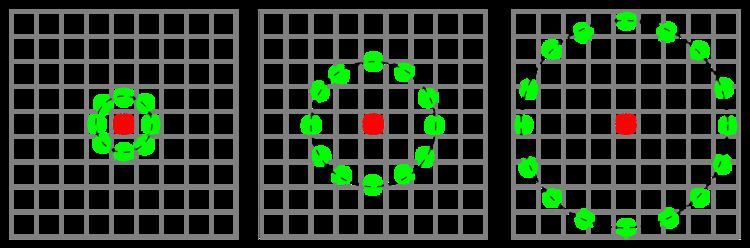# Local binary patterns

Updated onLocal binary patterns (LBP) is a type of visual descriptor used for classification in computer vision. LBP is the particular case of the Texture Spectrum model proposed in 1990. LBP was first described in 1994. It has since been found to be a powerful feature for texture classification; it has further been determined that when LBP is combined with the Histogram of oriented gradients (HOG) descriptor, it improves the detection performance considerably on some datasets. A comparison of several improvements of the original LBP in the field of background subtraction was made in 2015 by Silva et al. A full survey of the different versions of LBP can be found in Bouwmans et al.

## Concept

The LBP feature vector, in its simplest form, is created in the following manner:

• Divide the examined window into cells (e.g. 16x16 pixels for each cell).
• For each pixel in a cell, compare the pixel to each of its 8 neighbors (on its left-top, left-middle, left-bottom, right-top, etc.). Follow the pixels along a circle, i.e. clockwise or counter-clockwise.
• Where the center pixel's value is greater than the neighbor's value, write "0". Otherwise, write "1". This gives an 8-digit binary number (which is usually converted to decimal for convenience).
• Compute the histogram, over the cell, of the frequency of each "number" occurring (i.e., each combination of which pixels are smaller and which are greater than the center). This histogram can be seen as a 256-dimensional feature vector.
• Optionally normalize the histogram.
• Concatenate (normalized) histograms of all cells. This gives a feature vector for the entire window.
• The feature vector can now be processed using the Support vector machine or some other machine-learning algorithm to classify images. Such classifiers can be used for face recognition or texture analysis.

A useful extension to the original operator is the so-called uniform pattern, which can be used to reduce the length of the feature vector and implement a simple rotation invariant descriptor. This idea is motivated by the fact that some binary patterns occur more commonly in texture images than others. A local binary pattern is called uniform if the binary pattern contains at most two 0-1 or 1-0 transitions. For example, 00010000(2 transitions) is a uniform pattern, 01010100(6 transitions) is not. In the computation of the LBP histogram, the histogram has a separate bin for every uniform pattern, and all non-uniform patterns are assigned to a single bin. Using uniform patterns, the length of the feature vector for a single cell reduces from 256 to 59.

## Extensions

• Over-Complete Local Binary Patterns (OCLBP): OCLBP is a variant of LBP that has been shown to improve the overall performance on face verification . Unlike LBP, OCLBP adopts overlapping to adjacent blocks. Formally, the configuration of OCLBP is denoted as S : (a, b, v, h, p, r): an image is divided into a×b blocks with vertical overlap of v and horizontal overlap of h, and then uniform patterns LBP(u2,p,r) are extracted from all the blocks. Moreover, OCLBP is composed of several different configurations. For example, in their original paper, the authors used three configurations: S : (10,10,12,12,8,1),(14,14,12,12,8,2),(18,18,12,12,8,3). The three configurations consider three block sizes: 10×10, 14×14, 18×18, and half overlap rates along the vertical and horizontal directions. These configurations are concatenated to form a 40877 dimensional feature vector for an image of size 150x80.
• Transition Local Binary Patterns(tLBP): binary value of transition coded LBP is composed of neighbor pixel comparisons clockwise direction for all pixels except the central.
• Direction coded Local Binary Patterns(dLBP): the dLBP encodes the intensity variation along the four basic directions through the central pixel in two bits.
• Modified Local Binary Patterns(mLBP): the mLBP compares the values of neighboring pixels to the average of the intensity values in the 3x3 window.
• Multi-block LBP: the image is divided into many blocks, a LBP histogram is calculated for every block and concatenated as the final histogram.
• Volume Local Binary Pattern(VLBP): VLBP looks at dynamic texture as a set of volumes in the (X,Y,T) space where X and Y denote the spatial coordinates and T denotes the frame index. The neighborhood of a pixel is thus defined in three dimensional space, and volume textons can be extracted into histograms.
• RGB-LBP: This operator is obtained by computing LBP over all three channels of the RGB color space independently, and then concatenating the results together.
• ## Implementations

• CMV, includes the general LBP implementation and many further extensions over LBP histogram in MATLAB.
• Python mahotas, an open source computer vision package which includes an implementation of LBPs.
•  OpenCV's Cascade Classifiers support LBPs as of version 2.
• VLFeat, an open source computer vision library in C (with bindings to multiple languages including MATLAB) has an implementation.
• LBPLibrary is a collection of eleven Local Binary Patterns (LBP) algorithms developed for background subtraction problem. The algorithms were implemented in C++ based on OpenCV. A CMake file is provided and the library is complatible with Windows, Linux and Mac OS X. The library was tested successfully with OpenCV 2.4.10.
• BGSLibrary includes the original LBP implementation for motion detection as well as a new LBP operator variant combined with Markov Random Fields with improved recognition rates and robustness.
• dlib, an open source C++ library: implementation.
• ## References

Similar Topics
Tamsin Beaumont
Stuart Margolin
Joe Gallardo
Topics